# Vintage Bench-Top DC Hybrid Voltmeter

With a DC range of -25V to +25V, a voltmeter combining analog needle & digital LEDs to represent integer & fractional voltages respectively.

BeginnerFull instructions provided2 hours1,693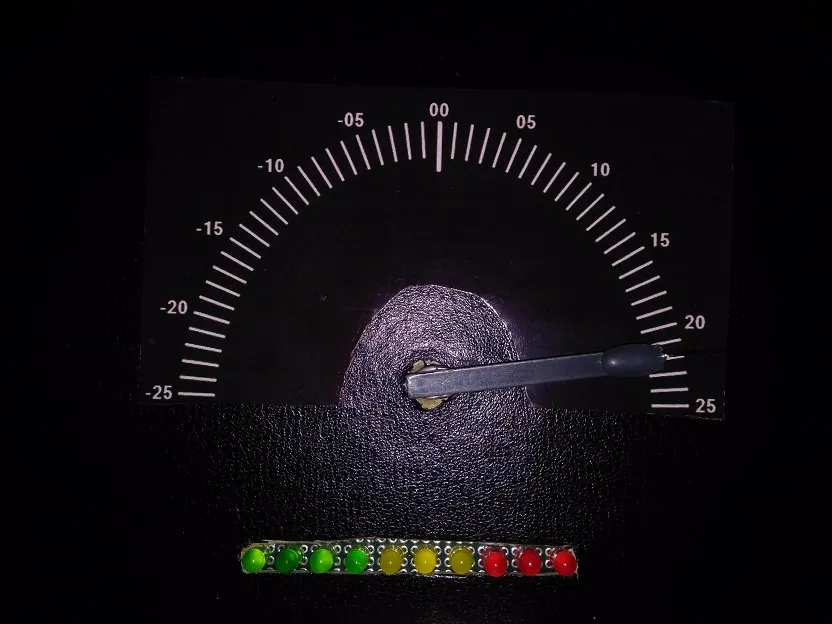## Things used in this project

### Hardware components

×1
 Servo Motor
×1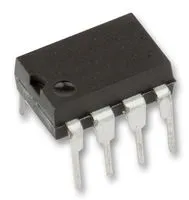Texas Instruments General Purpose Dual Op-Amp
×1
 SparkFun .1 uF Capacitor
×1
 Resistor 10 k
×1
 Resistor 1M
×2
 10 K Variable Multi Turn Pot
×1
 16 Mhz Xtal
×1×1
 Old Desktop Calendar
×1
 4AA Power Pack
×1
 SparkFun Jumper Wire M/F
×1
×1
 SparkFun 100uF Capacitor Electrolyte
×1
 10 uF Capacitor Electrolyte
×1
 SparkFun RGBY Mix LEDs
×1

### Software apps and online services

 Arduino IDE

### Hand tools and fabrication machines

 Hot glue gun

## Schematics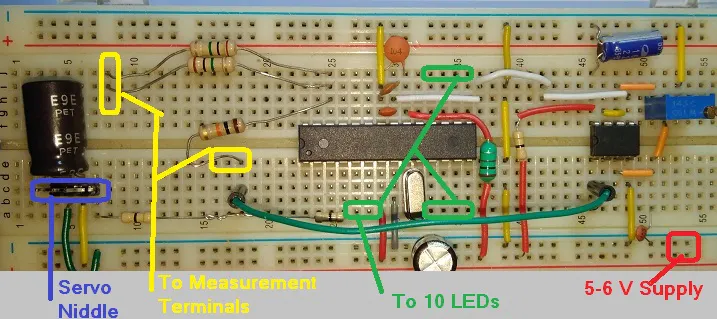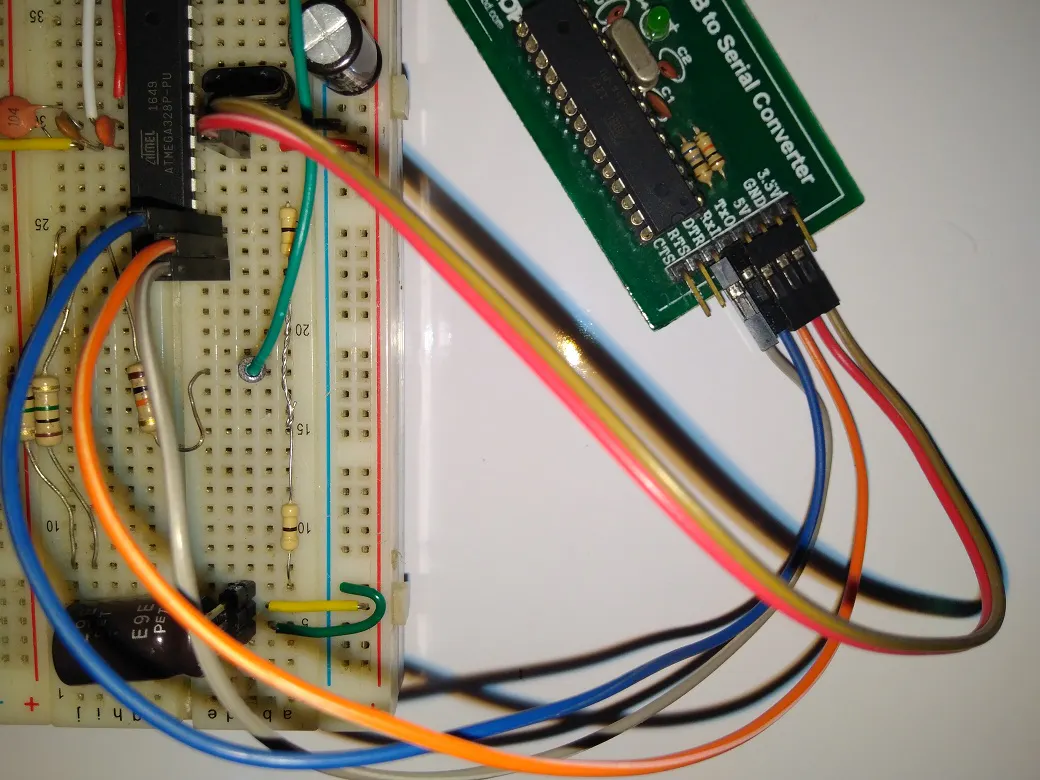### Dial 1

Black in White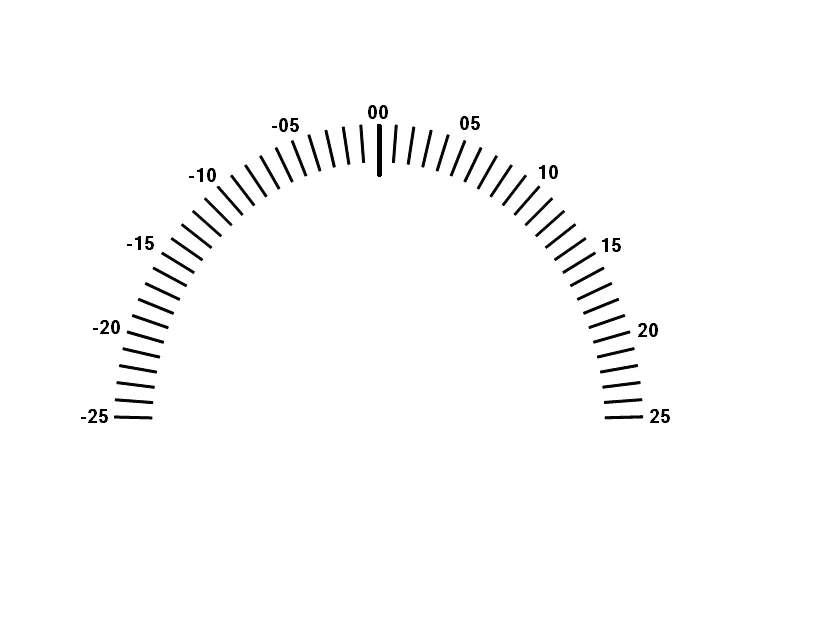### Dial 2

White in Black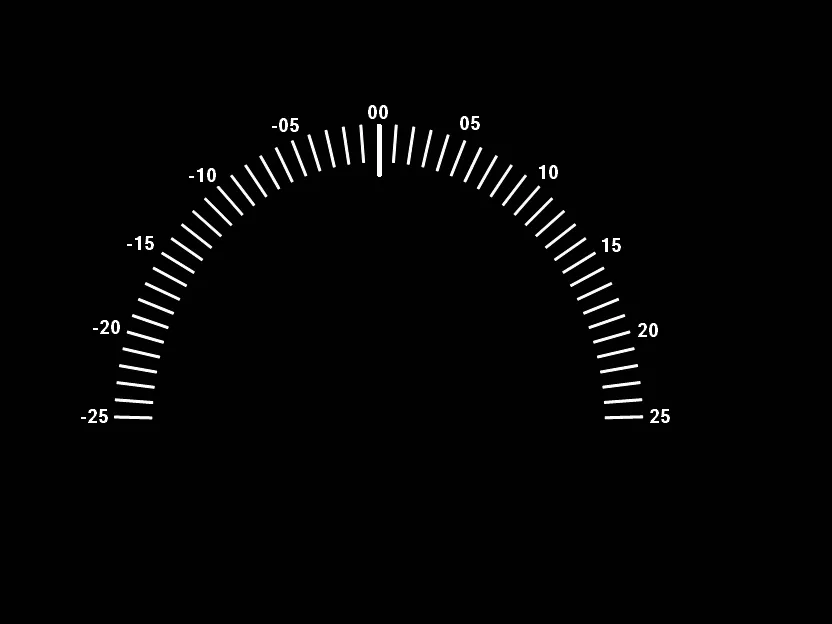### Atmega328P as Arduino Uno on Breadboard Pin Map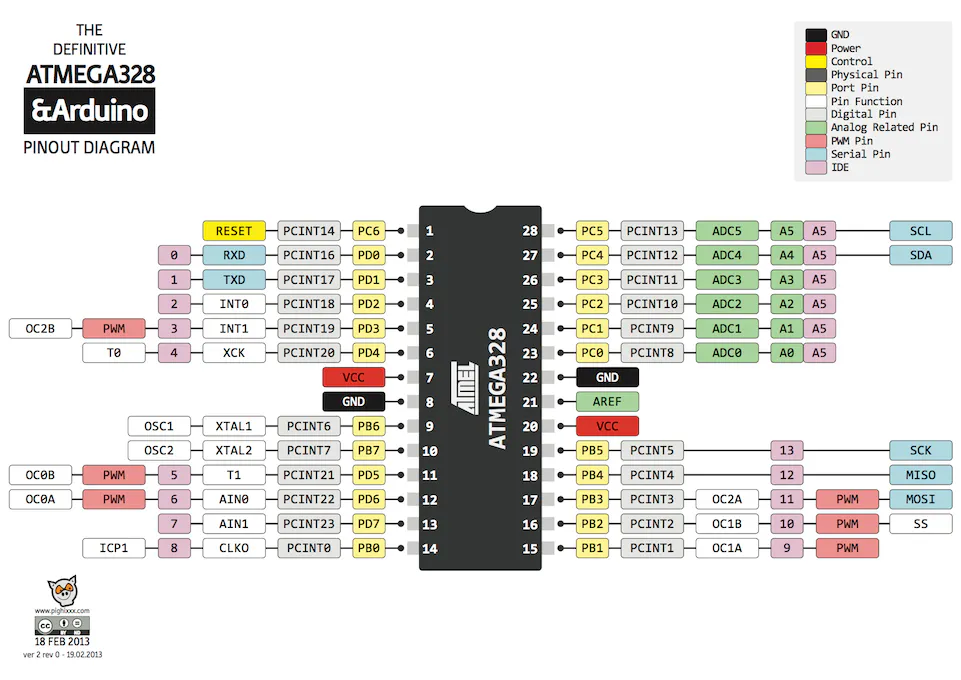## Code

### Sketch

Arduino
```#include <Servo.h>
# define AREF 1.08

float volt=0.0;
int offset=-3;
Servo needle;

void setup()
{
Servo_init();
LED_init();
needle_calibrate();
LED_test();
}

void loop()
{
for (int i=0;i<512;i++)
{
volt = volt+ 20*(x-512)*4.9*(AREF/2)/1023;

}
volt = volt/512;

if (volt<.09 & volt > 0.0)
{ volt = 0.0; } // rel (zeroing)

volt = volt - 0.03;// offset correction
if ( volt < 0.0 )
volt = volt - 0.19;

int pos;
pos = map(volt,-25,25,0,180);
needle.write(pos+offset);
LED_meter(volt); // glows LEDs according to fraction
delay(500);
volt=0.0;
}

{
analogReference(INTERNAL);  // set 1.1 v on AREF pin

}

void Servo_init(void)
{
needle.attach(2);  // attaches the servo on pin 2

}

void LED_init (void)
{
pinMode(3,1);
pinMode(4,1);
pinMode(5,1);
pinMode(6,1);
pinMode(7,1);
pinMode(8,1);
pinMode(9,1);
pinMode(10,1);
pinMode(11,1);
pinMode(12,1);
}

void needle_calibrate (void)
{

needle.write(0);
delay(1000);
needle.write(90);
delay(1000);
needle.write(180);
delay(1000);

}

void measure_volt (void)
{

}

void correct_volt (float v)
{

}

void volt_meter (void)
{

}

void LED_meter(float v)
{
int z = (v*10);
int y = v;
int x = z%y;
if (x==9)
{
digitalWrite (3,1);
digitalWrite (4,0);
digitalWrite (5,0);
digitalWrite (6,0);
digitalWrite (7,0);
digitalWrite (8,0);
digitalWrite (9,0);
digitalWrite (10,0);
digitalWrite (11,0);
digitalWrite (12,0);
}
if (x==8)
{
digitalWrite (3,1);
digitalWrite (4,1);
digitalWrite (5,0);
digitalWrite (6,0);
digitalWrite (7,0);
digitalWrite (8,0);
digitalWrite (9,0);
digitalWrite (10,0);
digitalWrite (11,0);
digitalWrite (12,0);
}

if (x==7)
{
digitalWrite (3,1);
digitalWrite (4,1);
digitalWrite (5,1);
digitalWrite (6,0);
digitalWrite (7,0);
digitalWrite (8,0);
digitalWrite (9,0);
digitalWrite (10,0);
digitalWrite (11,0);
digitalWrite (12,0);
}

if (x==6)
{
digitalWrite (3,1);
digitalWrite (4,1);
digitalWrite (5,1);
digitalWrite (6,1);
digitalWrite (7,0);
digitalWrite (8,0);
digitalWrite (9,0);
digitalWrite (10,0);
digitalWrite (11,0);
digitalWrite (12,0);
}
if (x==5)
{
digitalWrite (3,1);
digitalWrite (4,1);
digitalWrite (5,1);
digitalWrite (6,1);
digitalWrite (7,1);
digitalWrite (8,0);
digitalWrite (9,0);
digitalWrite (10,0);
digitalWrite (11,0);
digitalWrite (12,0);
}
if (x==4)
{
digitalWrite (3,1);
digitalWrite (4,1);
digitalWrite (5,1);
digitalWrite (6,1);
digitalWrite (7,1);
digitalWrite (8,1);
digitalWrite (9,0);
digitalWrite (10,0);
digitalWrite (11,0);
digitalWrite (12,0);
}

if (x==3)
{
digitalWrite (3,1);
digitalWrite (4,1);
digitalWrite (5,1);
digitalWrite (6,1);
digitalWrite (7,1);
digitalWrite (8,1);
digitalWrite (9,1);
digitalWrite (10,0);
digitalWrite (11,0);
digitalWrite (12,0);
}
if (x==2)
{
digitalWrite (3,1);
digitalWrite (4,1);
digitalWrite (5,1);
digitalWrite (6,1);
digitalWrite (7,1);
digitalWrite (8,1);
digitalWrite (9,1);
digitalWrite (10,1);
digitalWrite (11,0);
digitalWrite (12,0);
}
if (x==1)
{
digitalWrite (3,1);
digitalWrite (4,1);
digitalWrite (5,1);
digitalWrite (6,1);
digitalWrite (7,1);
digitalWrite (8,1);
digitalWrite (9,1);
digitalWrite (10,1);
digitalWrite (11,1);
digitalWrite (12,0);
}

}

void LED_test(void)
{

digitalWrite (3,0);
digitalWrite (4,0);
digitalWrite (5,0);
digitalWrite (6,0);
digitalWrite (7,0);
digitalWrite (8,0);
digitalWrite (9,0);
digitalWrite (10,0);
digitalWrite (11,0);
digitalWrite (12,0);
delay(400);
digitalWrite (3,1);
digitalWrite (4,1);
digitalWrite (5,1);
digitalWrite (6,1);
digitalWrite (7,1);
digitalWrite (8,1);
digitalWrite (9,1);
digitalWrite (10,1);
digitalWrite (11,1);
digitalWrite (12,1);
delay(200);

}
```

## Credits

### Shahariar

48 projects • 158 followers
"What Kills a 'Great life' is a 'Good Life', which is Living a Life Inside While Loop"